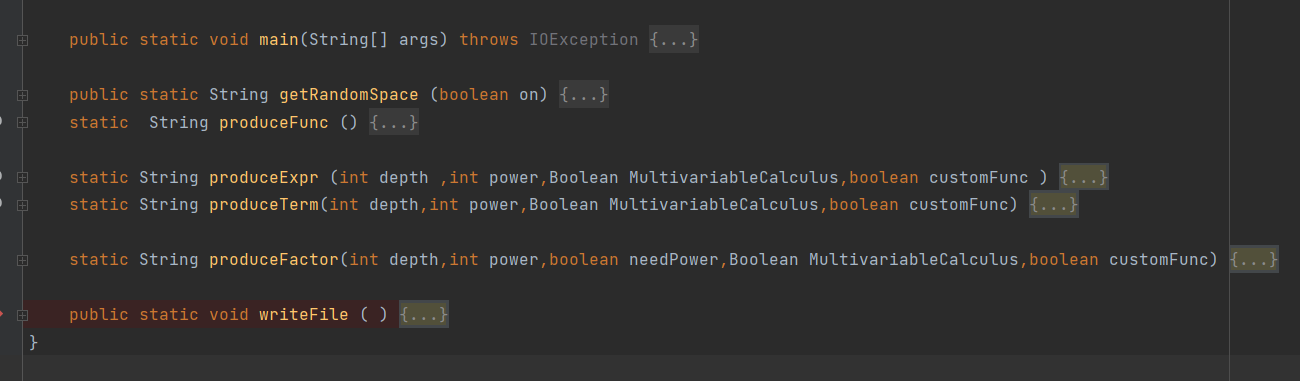# 20373222李世昱第四单元总结

### 四个单元的演进

##### 一单元：1
f(x,y,z)=-+(---0)0
-+-0*sum(i,1,10,x
2)-+(--+2+++0-0)1
2
f(x,y,z)=-+y
1
z1-+0*x2
g(x,y,z)=-++2z**0
++(-+-0
2)-0
3
f(x,y,z)=-0
g(x,y,z)=+-1--0
h(x,y,z)=+-y**2
1++-0
--cos(sin((-+x1-++0*x1)))--(--x**1+--4)

### 几个建议

posted @ 2022-06-22 00:23  zdfwqc  阅读(31)  评论(0编辑  收藏  举报